# 计数器的设计

## 预习报告

### 内容一

#### JK 触发器功能/真值表

CP 时钟 J K 工作状态 $Q^+$
$\downarrow$ 0 0 保持 Q
$\downarrow$ 0 1 置零 0
$\downarrow$ 1 0 置一 1
$\downarrow$ 1 1 翻转 $\overline Q$

#### 用 Proteus 设计电路，并运行仿真

$0000\to1111\to1110\to1101\to1100\to1011\to1010\to1001\to1000\to0111\to0110\to0101\to0100\to0011\to0010\to0001\to0000\to\dots$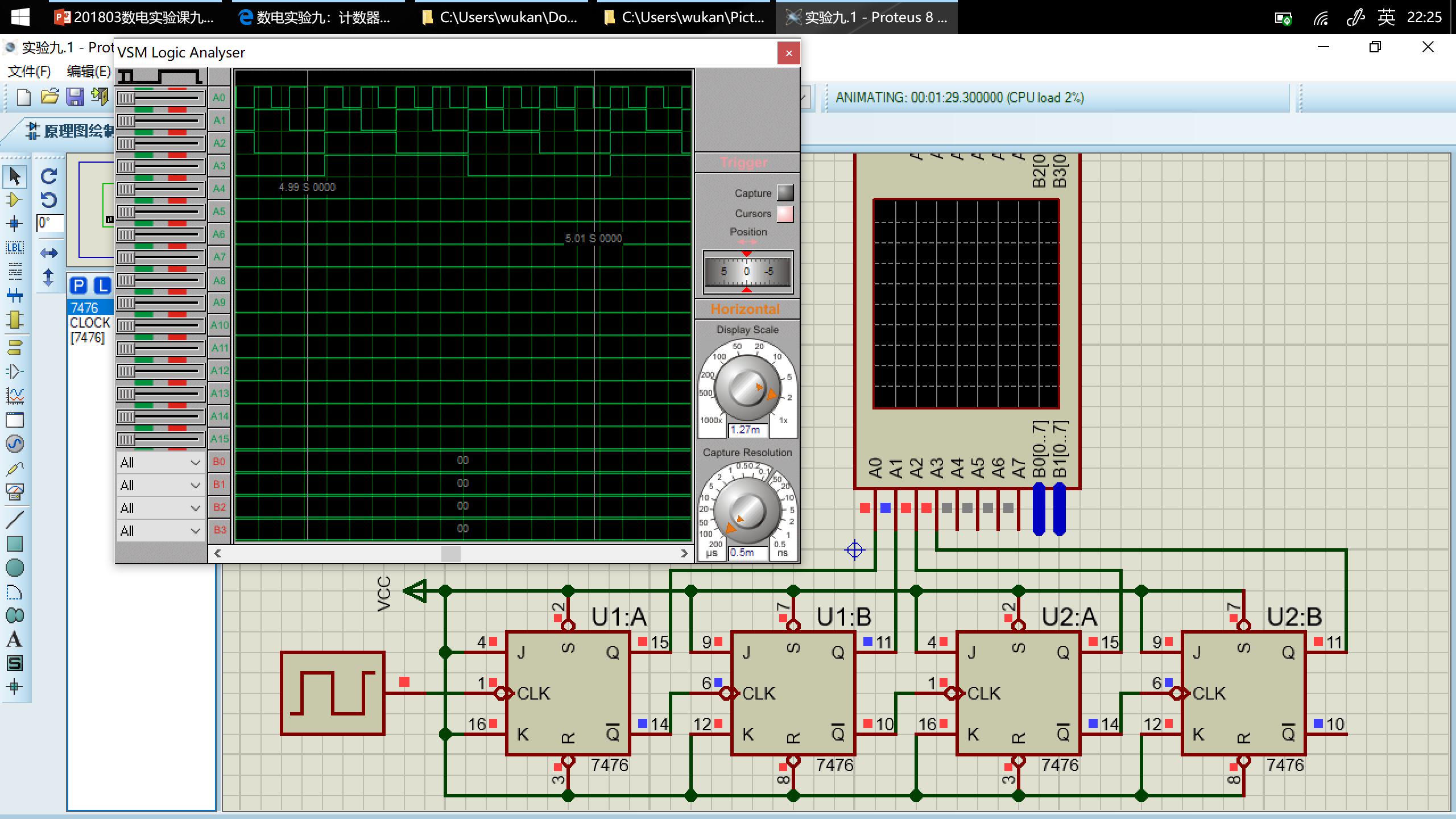### 内容二

#### 用 Proteus 设计电路，并运行仿真

$0000\to0001\to0010\to0011\to0100\to0101\to0110\to0111\to1000\to1001\to1010\to1011\to1100\to1101\to1110\to1111\to0000\dots$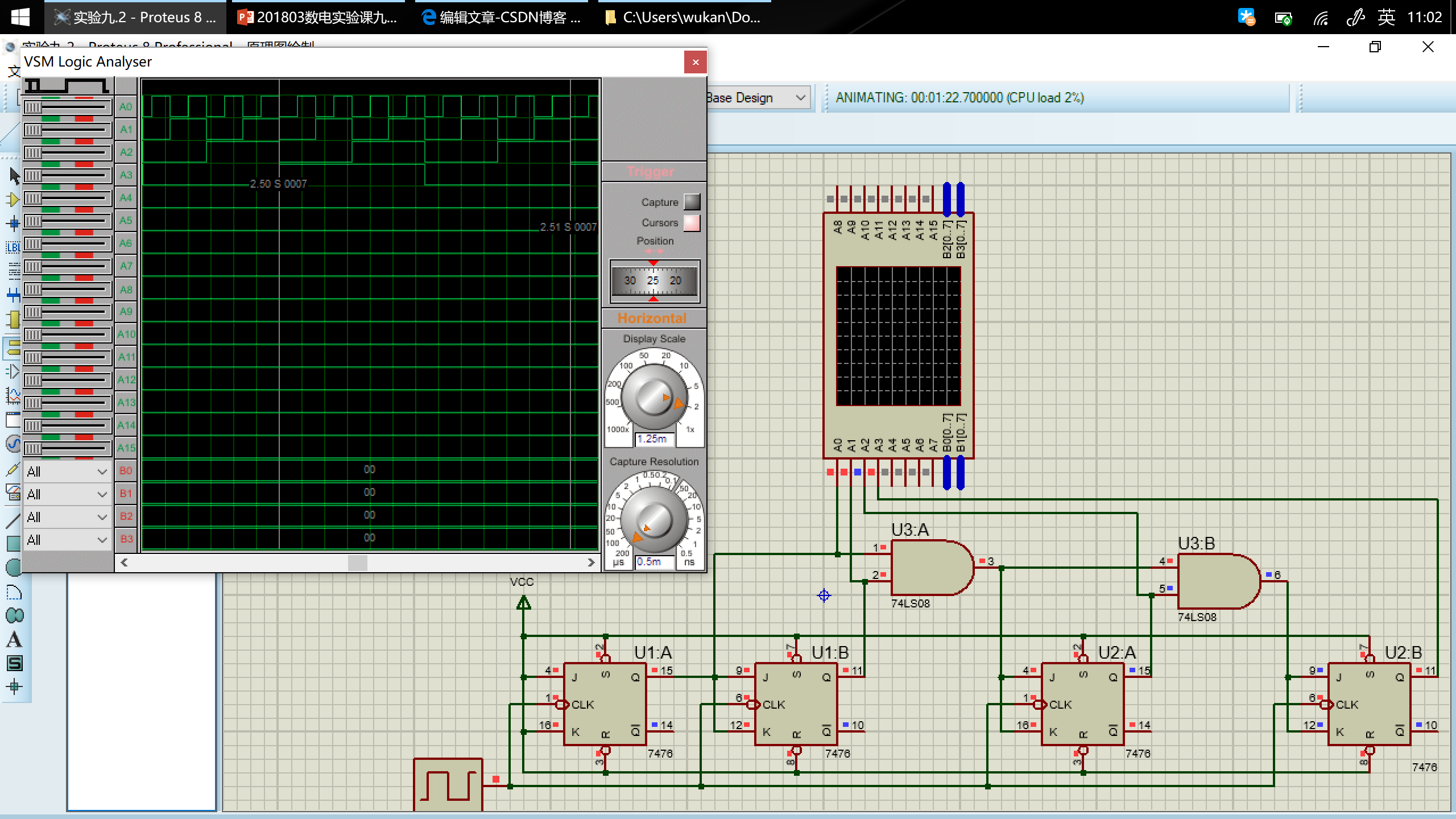### 内容三

#### 74LS194 功能/真值表

CP 时钟 $\overline{Cr}$清零 ${S_1}$ ${S_0}$ 工作状态 $Q_A^+$ $Q_B^+$ $Q_C^+$ $Q_D^+$
$\uparrow$ 0 X X 置零 0 0 0 0
$\uparrow$ 1 0 0 保持 $Q_A$ $Q_B$ $Q_C$ $Q_D$
$\uparrow$ 1 0 1 右移 $D_{SR}$ $Q_A$ $Q_B$ $Q_C$
$\uparrow$ 1 1 0 左移 $Q_B$ $Q_C$ $Q_D$ $D_{SL}$
$\uparrow$ 1 1 1 并行送数 $D_0$ $D_1$ $D_2$ $D_3$

#### 用 Proteus 设计电路，并运行仿真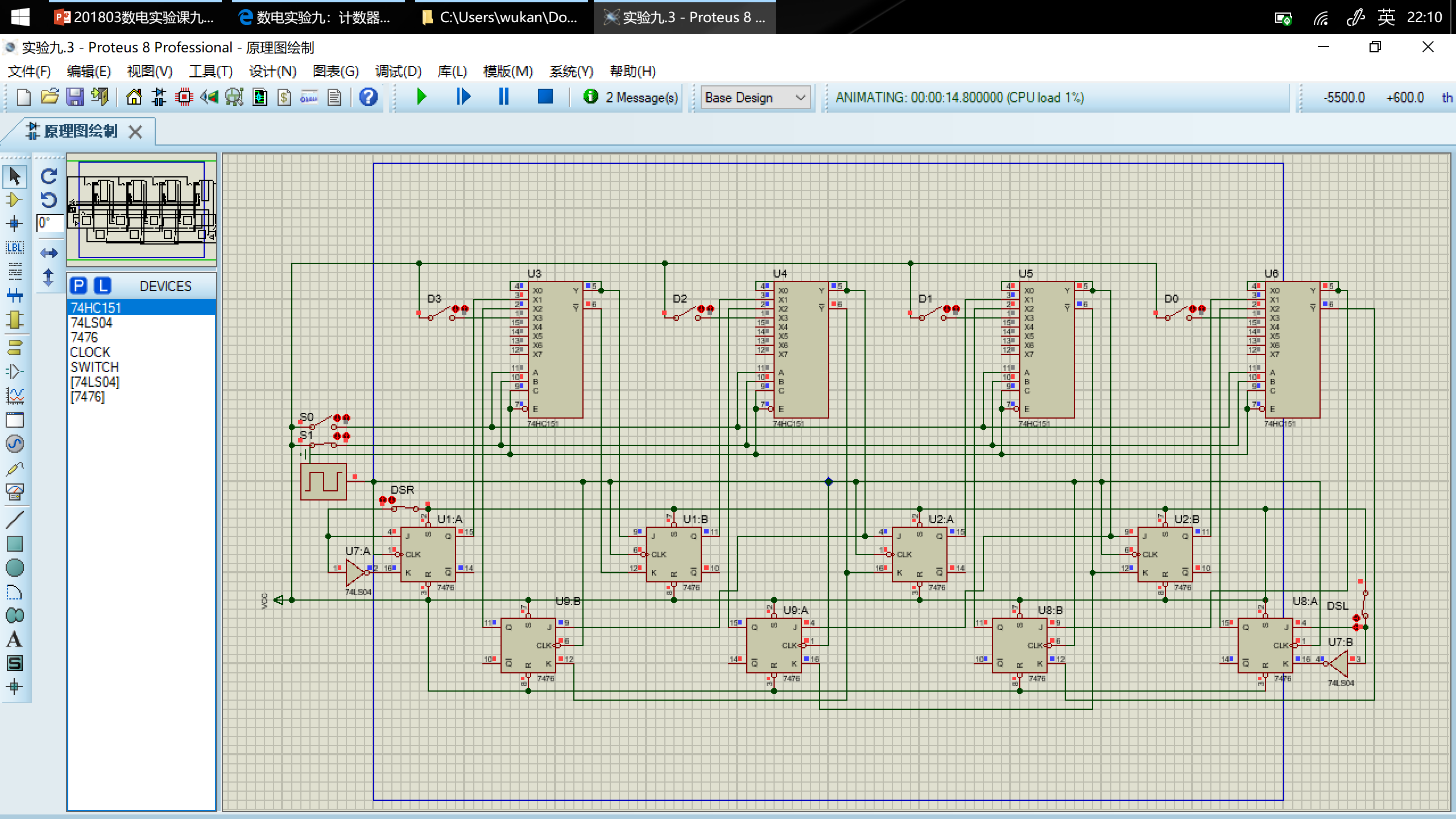### 内容四

$0001\to0010\to0011\to0100\to0101\to0110\to0111\to1000\to1001\to1010\to1011\to1100\to0001\to\dots$

#### 次态卡诺图

$Q_3^+Q_2^+\setminus Q_1^+Q_0^+$ 00 01 11 10
00 xxxx 0010 0100 0011
01 0101 0110 1000 0111
11 0001 xxxx xxxx xxxx
10 1001 1010 1100 1011

#### 卡诺图化简得到每个触发器方程

$Q_3^+Q_2^+\setminus Q_1^+Q_0^+$ 00 01 11 10
00 x 0 0 0
01 0 0 1 0
11 0 x x x
10 1 1 1 1

$Q_3^+=Q_3\overline{Q_2}+\overline{Q_3}Q_2Q_1Q_0$

$Q_3^+Q_2^+\setminus Q_1^+Q_0^+$ 00 01 11 10
00 x 0 1 0
01 1 1 0 1
11 0 x x x
10 0 0 1 0

$Q_2^+=\overline{Q_3}Q_2\overline{Q_1}+\overline{Q_2}Q_1Q_0+\overline{Q_3}Q_2\overline{Q_0}$

$Q_3^+Q_2^+\setminus Q_1^+Q_0^+$ 00 01 11 10
00 x 1 0 1
01 0 1 0 1
11 0 x x x
10 0 1 0 1

$Q_1^+=Q_1\overline{Q_0}+\overline{Q_1}Q_0$

$Q_3^+Q_2^+\setminus Q_1^+Q_0^+$ 00 01 11 10
00 x 0 0 1
01 1 0 0 1
11 1 x x x
10 1 0 0 1

$Q_0^+=\overline{Q_0}$

#### 驱动器方程

$J_3=Q_2Q_1Q_0,K_3=Q_2$

$J_2=Q_1Q_0,K_2=\overline{\overline{Q_3}(\overline{Q_1}+\overline{Q_0})}=Q_3+Q_1Q_0$

$J_1=K_1=Q_0$

$J_0=K_0=1$

#### 用 Proteus 设计电路，并运行仿真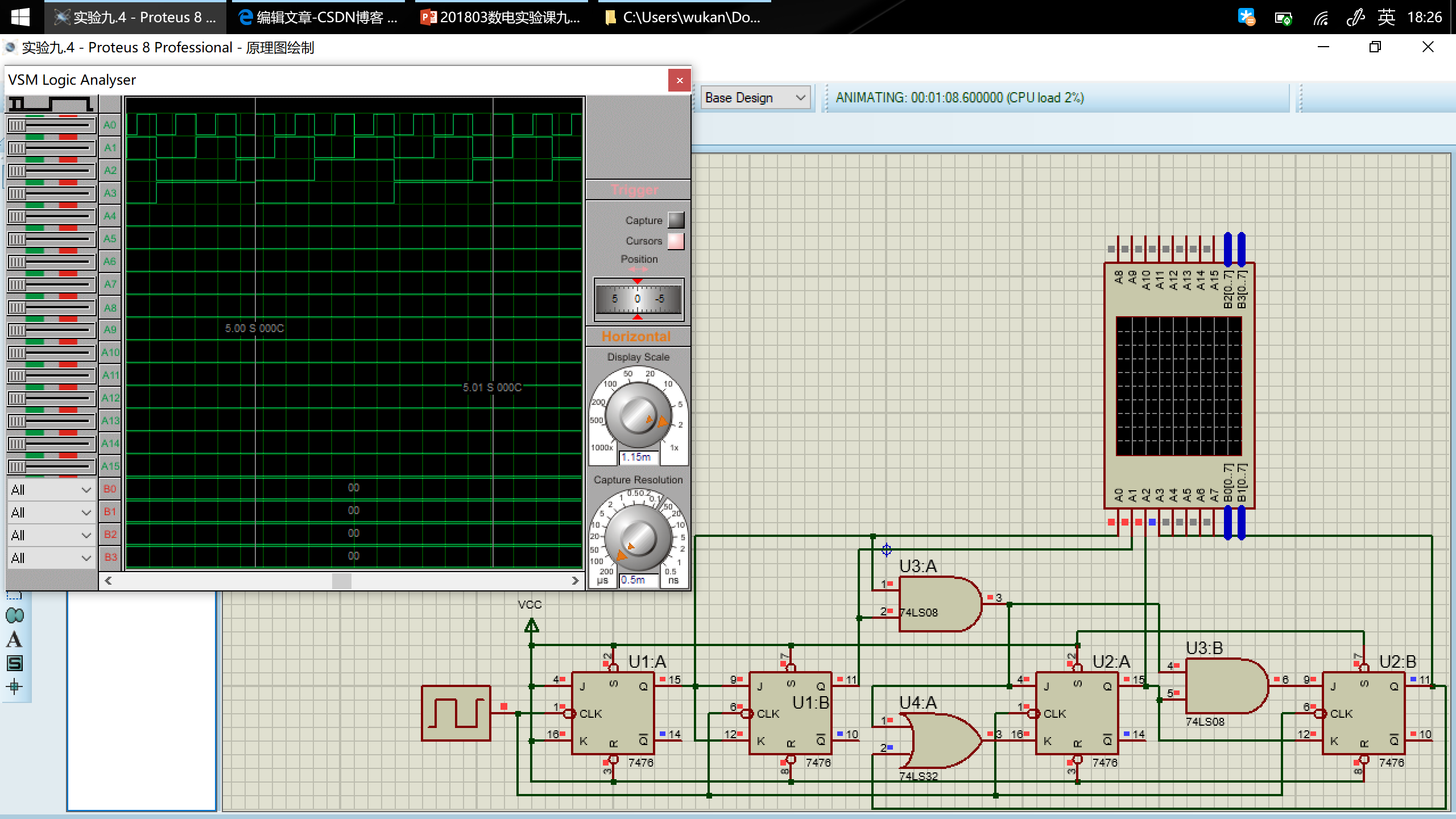### 内容五

#### 次态卡诺图

$Q_3^+Q_2^+\setminus Q_1^+Q_0^+$ 00 01 11 10
00 xxxx 1100 0010 0001
01 0011 0100 0110 0101
11 1011 xxxx xxxx xxxx
10 0111 1000 1010 1001

#### 卡诺图化简得到每个触发器方程

$Q_3^+Q_2^+\setminus Q_1^+Q_0^+$ 00 01 11 10
00 x 1 0 0
01 0 0 0 0
11 1 x x x
10 0 1 1 1

$Q_3^+=Q_3Q_2+Q_3Q_1+Q_3Q_0+\overline{Q_3}\,\overline{Q_2}\,\overline{Q_1}$

$Q_3^+Q_2^+\setminus Q_1^+Q_0^+$ 00 01 11 10
00 x 1 0 0
01 0 1 1 1
11 0 x x x
10 1 0 0 0

$Q_2^+=\overline{Q_3}\,\overline{Q_2}\,\overline{Q_1}+\overline{Q_2}\,\overline{Q_1}\,\overline{Q_0}+Q_2Q_1+Q_2Q_0$

$Q_3^+Q_2^+\setminus Q_1^+Q_0^+$ 00 01 11 10
00 x 0 1 0
01 1 0 1 0
11 1 x x x
10 1 0 1 0

$Q_1^+=Q_1Q_0+\overline{Q_1}\,\overline{Q_0}$

$Q_3^+Q_2^+\setminus Q_1^+Q_0^+$ 00 01 11 10
00 x 0 0 1
01 1 0 0 1
11 1 x x x
10 1 0 0 1

$Q_0^+=\overline{Q_0}$

#### 驱动方程

$J_3=\overline{Q_2}\,\overline{Q_1},K_3=\overline{Q_2+Q_1+Q_0}=\overline{Q_2}\,\overline{Q_1}\,\overline{Q_0}$

$J_2=\overline{Q_1}\,\overline{Q_3}+\overline{Q_1}\,\overline{Q_0},K_2=\overline{Q_1+Q_0}=\overline{Q_1}\,\overline{Q_0}$

$J_1=K_1=\overline{Q_0}$

$J_0=K_0=1$

#### 用 Proteus 设计电路，并运行仿真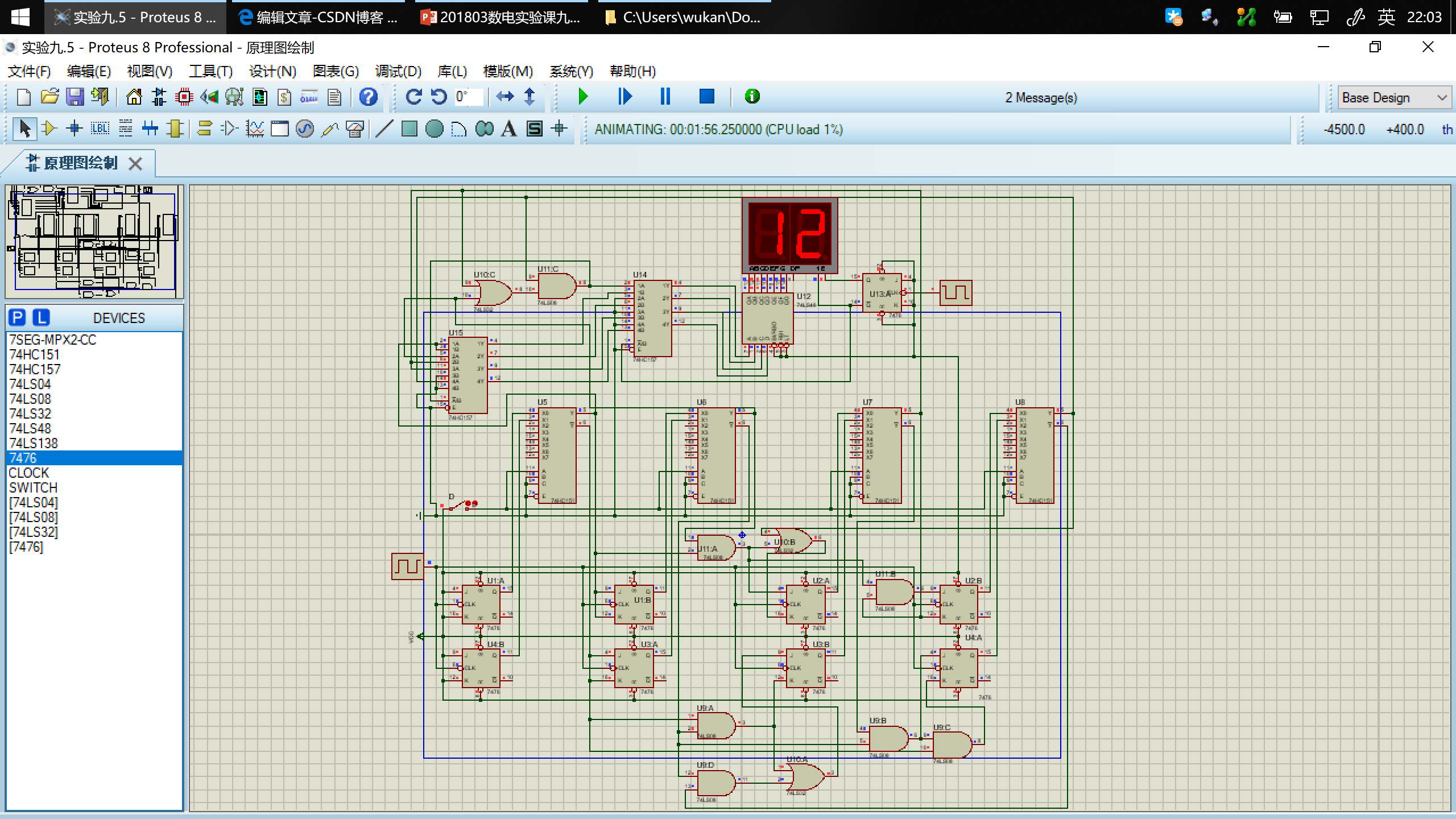#### 用 Vivado 设计电路，并烧写到 Basys3 实验板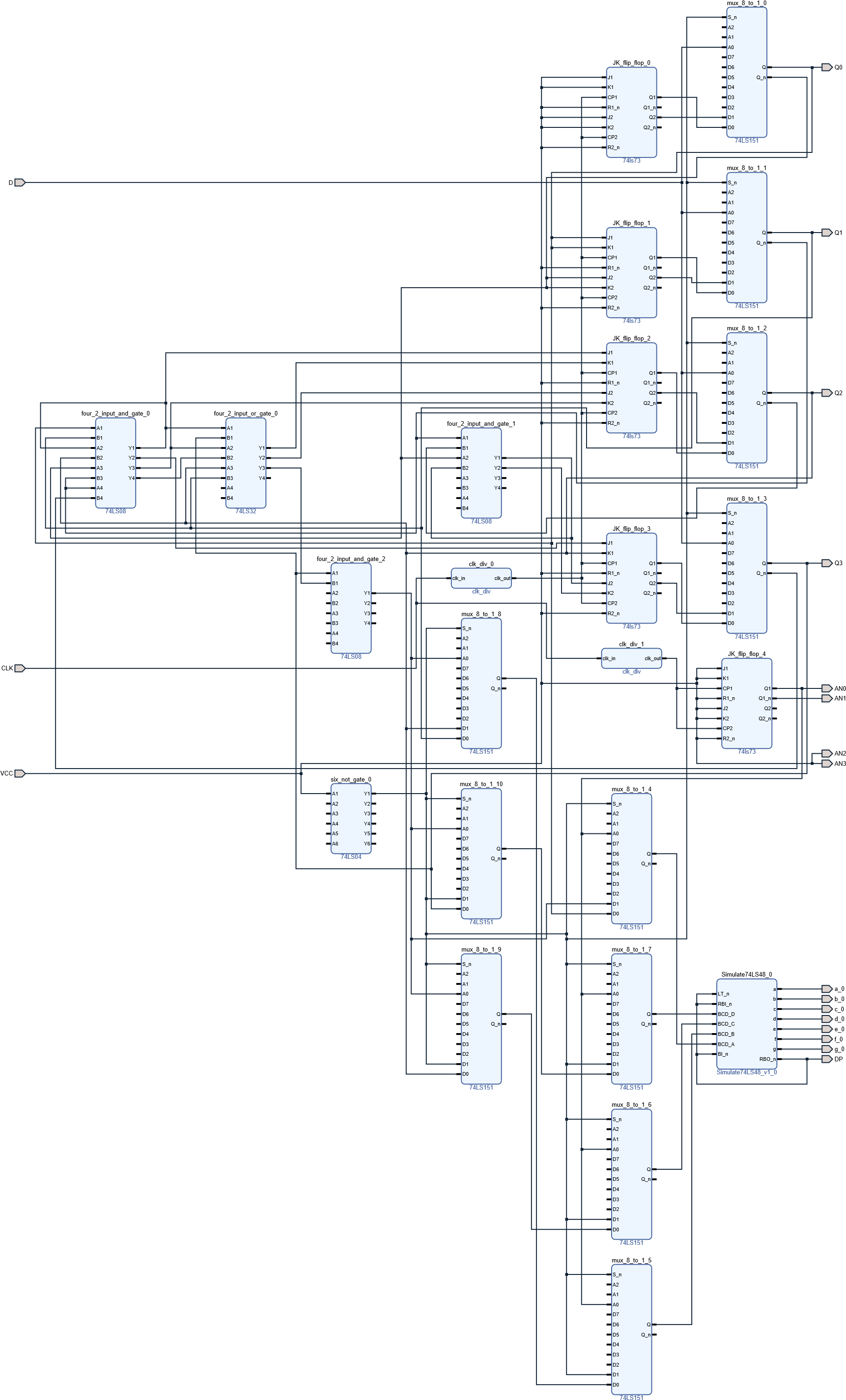##### 端口映射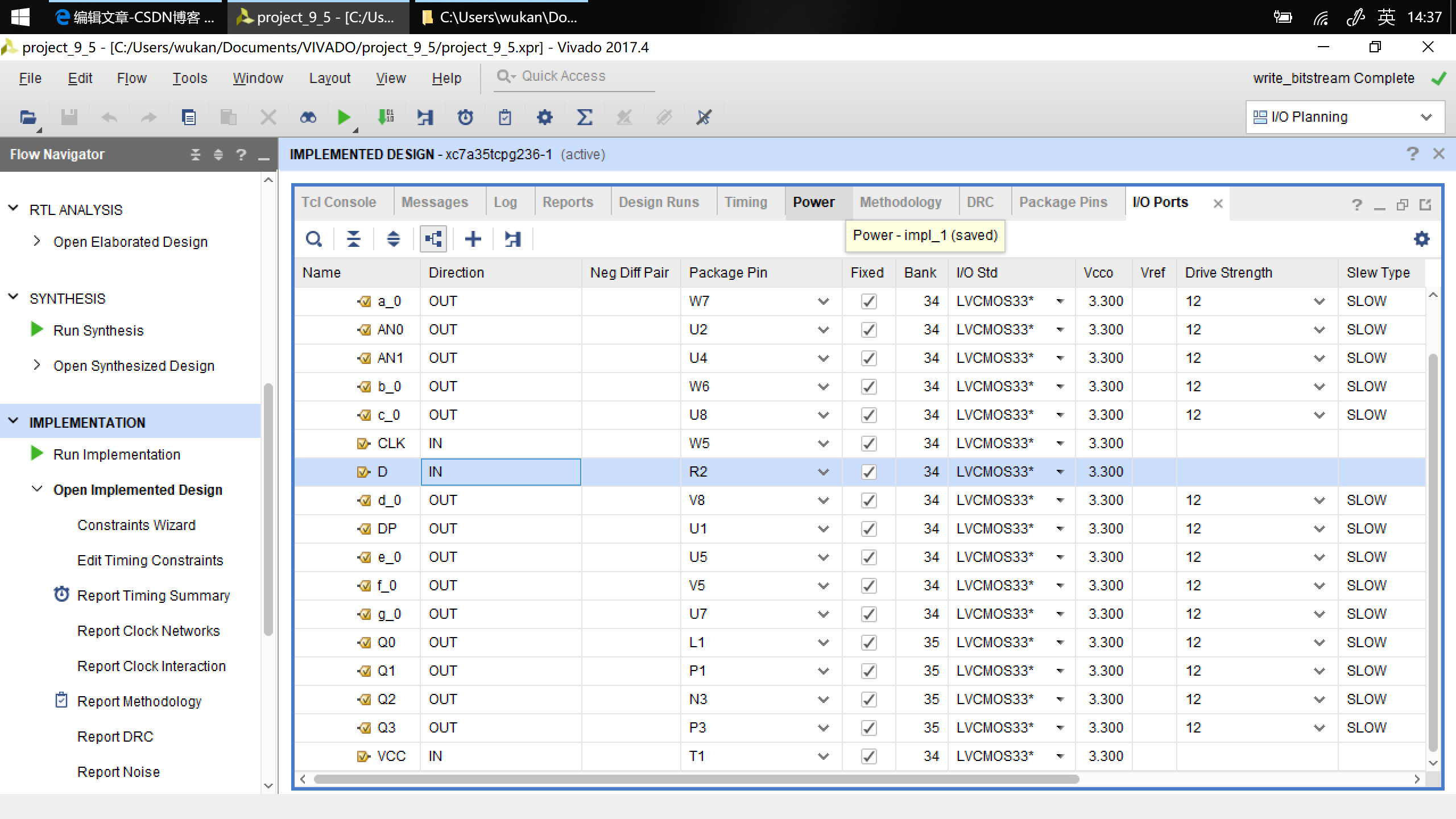##### 烧写到 Basys3 实验板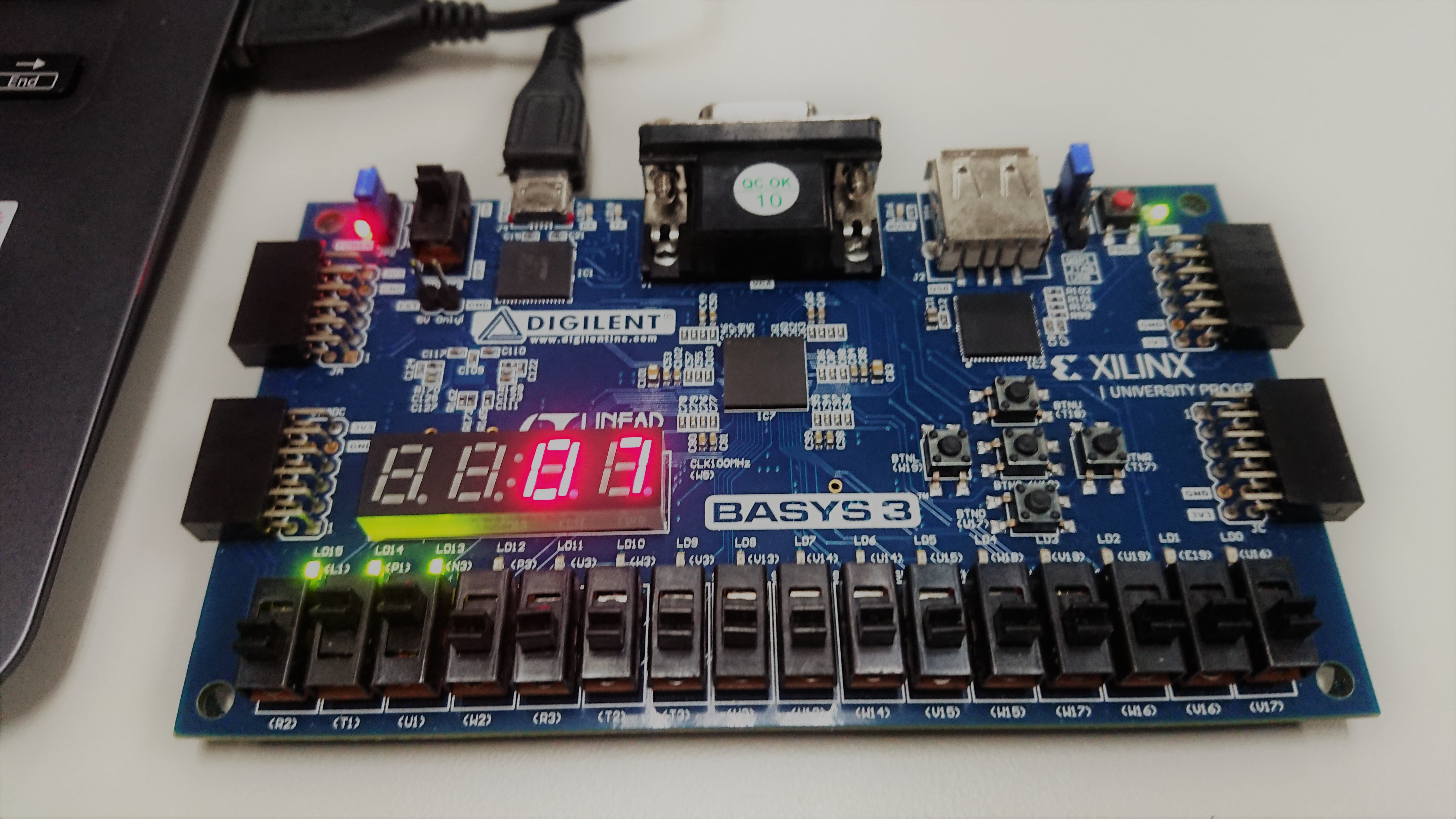## 实验报告

### 内容四

#### 转换电路的效果检验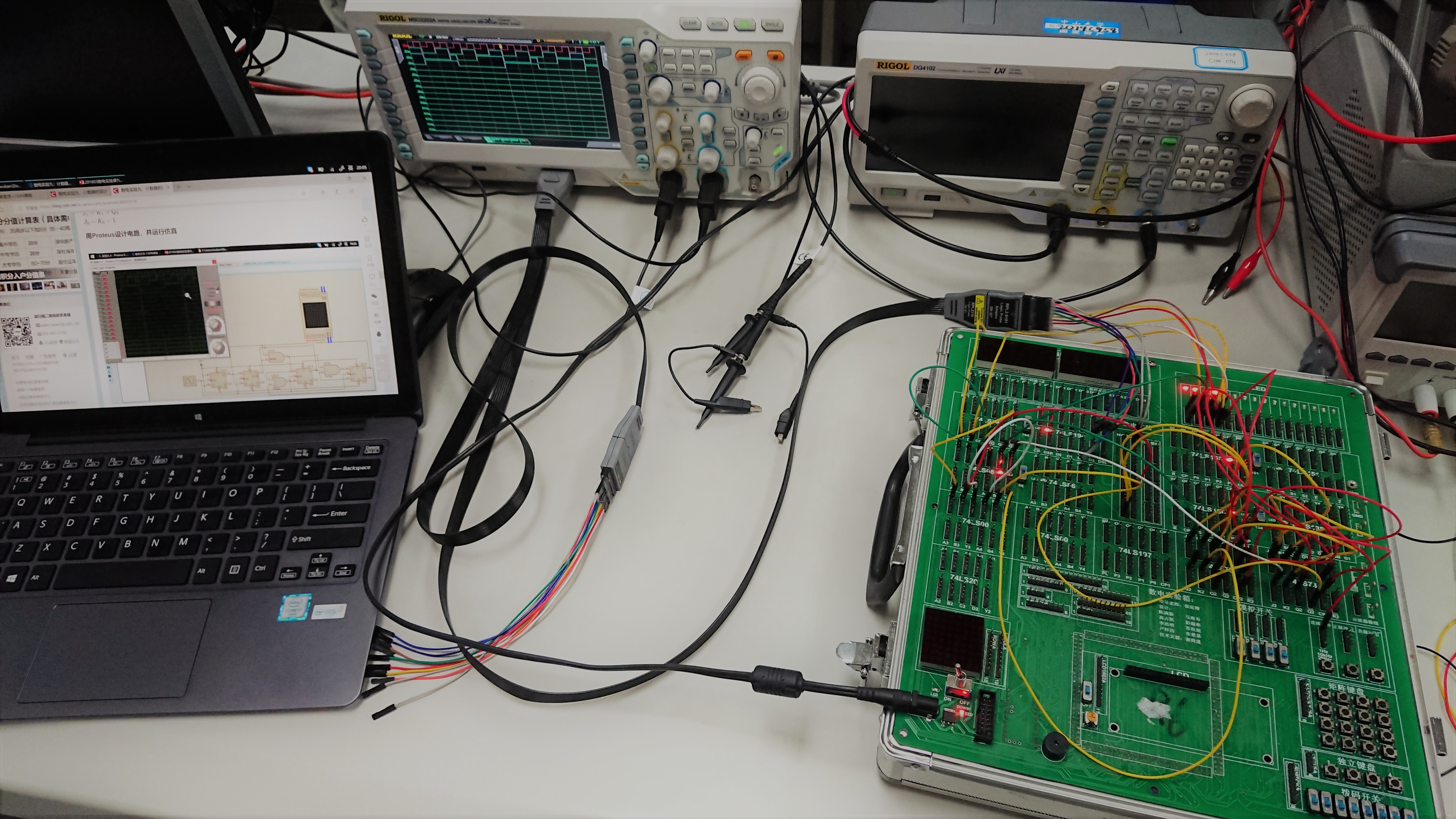#### 波形分析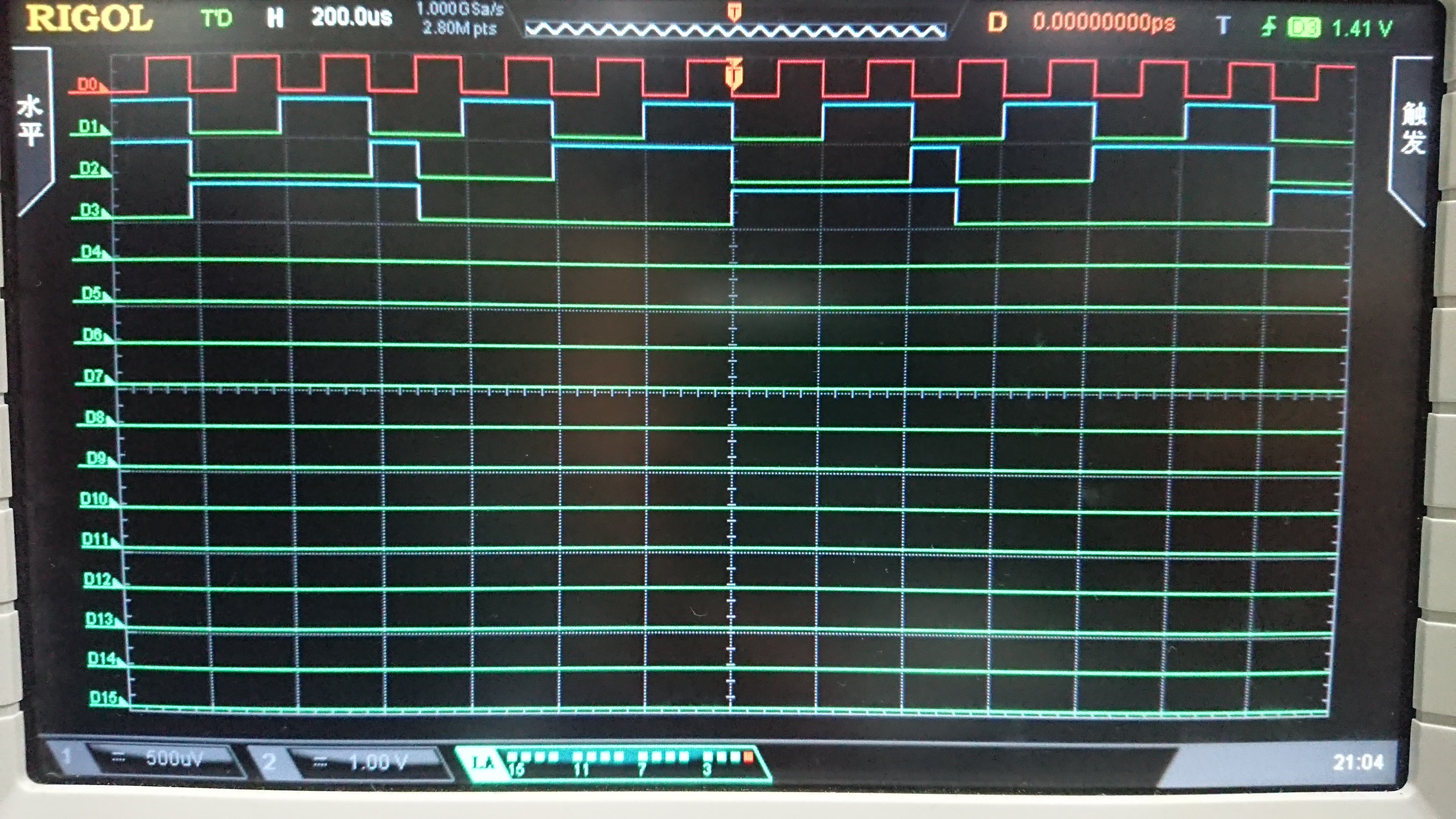### 实验心得和体会

1. 通过本次实验，我了解了 portuse 仿真软件上开关的使用。
2. 通过本次实验，我熟悉了时序逻辑电路的分析步骤和方法。
3. 通过本次实验，我更熟悉了示波器的使用。
4. 通过本次实验，我提高了对错误电路的调试能力。
5. 通过本次实验，我提高了电路的推导和检查能力。